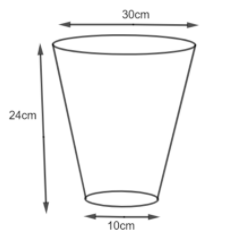Courses
Courses for Kids
Free study material
Free LIVE classes
MoreLIVE
Join Vedantu’s FREE Mastercalss

# A bucket open at the top, and make up of a metal sheet is in the form of a frustum of a cone. The depth of the bucket is 24 cm and the diameters of its upper and lower circular ends are 30 cm and 10 cm respectively. Find the cost of the metal sheet used in it at the rate of Rs. 10 per $100c{{m}^{2}}.\left[ \text{Use }\pi =3.14 \right]$Verified
334.8k+ views
Hint: We are given a bucket in the form of a frustum. We have the upper and lower diameter. We first have to find the radius and then find the curved surface area of the frustum as $S=\pi \left( R+r \right)l$ where l is the slant height. We can find the slant height (l) using the formula $l=\sqrt{{{h}^{2}}+{{\left( R-r \right)}^{2}}}$ and then we will find the area and then the total cost by multiplying the area by cost per $c{{m}^{2}}.$

We are given a bucket in the shape of the frustum with the height as 24 cm while the upper circular part that is the diameter is 30 cm while the lower circular part diameter is 10 cm.So, at first, we will find the radius of the upper and lower circular end and then find the surface area. We are given that the upper diameter is 30cm. Therefore, we know that
$R=\dfrac{D}{2}$
Substituting the value of D in the above formula, we get,
$\Rightarrow R=\dfrac{30}{2}=15cm$
Therefore, the upper radius, R = 15 cm.
Similarly, the lower diameter is 10 cm. So, we know that
$r=\dfrac{d}{2}$
$\Rightarrow r=\dfrac{10}{2}=5cm$
Therefore, the lower radius = 5 cm.
So, we have the following details about the frustum.
Height (h) = 24 cm
Upper Circular Radius (R) = 15 cm
Lower Circular Radius (r) = 5 cm
To find the cost of the metal sheet, we have to find the total area of the metal sheet. So,
The total area of the metal sheet = Curved Surface Area of the frustum + Area of the lower circle
$=\pi \left( r+R \right)l+\pi {{r}^{2}}$
First, we will find l and then use the above formula. We know that the slant height (l) of the frustum is given as,
$l=\sqrt{{{h}^{2}}+{{\left( R-r \right)}^{2}}}$
Here, h = 24, R = 15, r = 5. So,
$l=\sqrt{{{\left( 24 \right)}^{2}}+{{\left( 15-5 \right)}^{2}}}$
$\Rightarrow l=\sqrt{{{24}^{2}}+{{10}^{2}}}$
$\Rightarrow l=\sqrt{576+100}$
$\Rightarrow l=\sqrt{676}$
$\Rightarrow l=\sqrt{{{26}^{2}}}$
Therefore, we get l = 26 cm.
Now, we have all the required details to find the total area of the metal sheet.
$\Rightarrow \text{Total Area}=3.14\left( 15+5 \right)26+3.14\times 25$
$\Rightarrow \text{Total Area}=3.14\times 20\times 26+3.14\times 25$
After solving, we will get,
$\Rightarrow \text{Total Area}=1711.3c{{m}^{2}}$
Now, we are given that $100c{{m}^{2}}$ of the metal cost Rs. 10 that means,
$100c{{m}^{2}}=Rs.10$
Using the unitary method, we will get,
$1c{{m}^{2}}=\dfrac{Rs.10}{100}$
$1c{{m}^{2}}=\dfrac{Rs.1}{10}$
Now, we will find the cost of $1711.3c{{m}^{2}}$ metal sheet.
$\Rightarrow 1711.3c{{m}^{2}}=\dfrac{1}{10}\times 1711.3$$\Rightarrow 1711.3c{{m}^{2}}=Rs.171.13$
So, we get the cost of preparing the bucket with the metal sheet as Rs. 171.13.

Note: The curved surface area of the frustum has slant height in its formula. So, students should not take H as l and solve the solution. It will lead to the wrong solution. First students need to find l using the formula $l=\sqrt{{{h}^{2}}+{{\left( R-r \right)}^{2}}}$ and then use that l to solve the frustum. The conversion of rate into cost per $c{{m}^{2}}$ is necessary, then only we can find the cost of $1711.3c{{m}^{2}}$ of the metal.
Last updated date: 25th Sep 2023
Total views: 334.8k
Views today: 4.34k